• 遗憾的是，饼图是一种应用场合很有限的图表类型，图表创建者很容易将饼图绘制得难以看懂。如果你要使用饼图，请尝试遵守下面的规则： 确保你关心的是相对频率。每个扇区应该表示整体的一部分，而不是单独的数值...


文章目录
饼图直方图其他方法

饼图
饼图是一种常见的单变量图表，用于描绘分类变量各类别的相对频率。饼图中的扇区代表频率大小；角度或面积越大，该类别就出现地越频繁。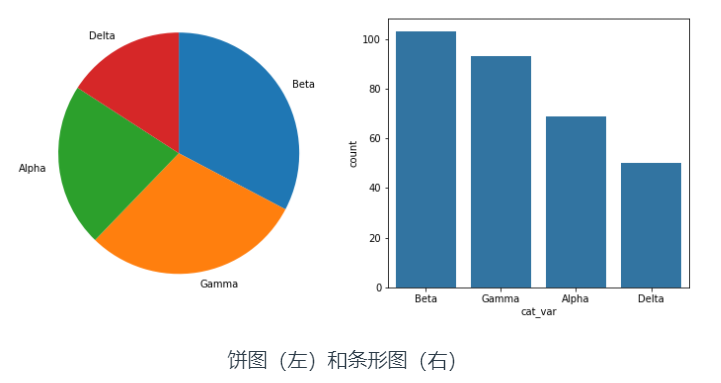遗憾的是，饼图是一种应用场合很有限的图表类型，图表创建者很容易将饼图绘制得难以看懂。如果你要使用饼图，请尝试遵守下面的规则：
确保你关心的是相对频率。每个扇区应该表示整体的一部分，而不是单独的数值（除非变量能够求和成某个整体）。将扇区限制在一定数量内。饼图最好只包含两到三个扇区，如果扇区足以明确区分，也可以包含四到五个。如果你有很多个类别，并且某些类别所占的比例很小，那可以将它们组合到一起，或者将这些比例很小的类别放到 “其他” 类别中。系统地绘制数据。绘制饼图的一种常见方法是从圆圈的顶部开始，然后沿着顺时针方向绘制每个分类级别，从最常见的到最不常见的排列。如果有三个类别，并且想要对比其中两个，一种常见绘制方法是将这两个类别放在 12 点钟方向的两侧，第三个类别填充在底部剩余部分。
如果无法满足这些规则，则建议使用条形图。通常选择条形图更保险。长条高度比面积或角度更精确，并且条形图比饼图更紧凑。对于值很多的变量来说，条形图更灵活。
你可以使用 matplotlib 的 pie 函数创建饼图。此函数需要数据为汇总的形式：函数的主要参数是扇区大小 。
# code for the pie chart seen above
sorted_counts = df['cat_var'].value_counts()
plt.pie(sorted_counts, labels = sorted_counts.index, startangle = 90,
counterclock = False);
plt.axis('square')

为了遵守上面提到的规则，我们可以添加 “startangle = 90” 和 "counterclock = False"这两个参数，在垂直上方开始第一个扇区，然后按照顺时针方向有序地绘制每个扇区。axis 函数的使用和 "square" 参数的设定， 将使 x 和 y 轴的长度相等。不调用此函数的话，最终的图形可能看起来更像椭圆而不是正圆。
饼图的一种变体形式是环形图。它看起来很像饼图，但是图形中间有个洞。从视觉感知上来看，环形图和饼图没有什么太大的区别，所以应该按照饼图的相同规则使用环形图。选择饼图还是环形图的原因之一可能是看起来是否美观。比如，你可能会在一些报告中看到，在环形图中间的空白处展示一些统计数据，充分利用空间。
要创建环形图，可以在 pie 函数调用中添加 "wedgeprops" 参数。默认情况下，饼图（圆形）的半径是 1；将扇区宽度属性设为小于 1 的值会删除圆形中心的颜色。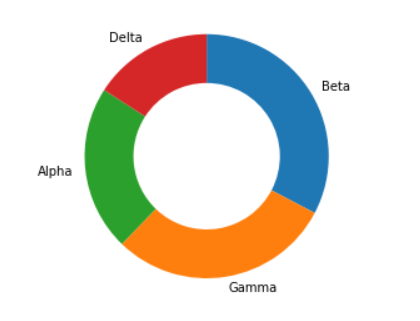直方图
直方图用来绘制数值变量的分布情况。它是条形图的数值变量版本。但是，我们不会为每个单独的数值绘制一个长条，而是定义几个连续的分组（bin），为每个分组绘制长条以代表相应的数字。例如，使用 matplotlib 的 hist 函数的默认设置：
plt.hist(data = df, x = 'num_var')

可以看出，最左侧的分组（约为 0 到 2.5 之间）包含 8 个数据点，其相邻的分组 （约为 2.5 到 5 之间）包含 9 个数据点。总体来说，可以看出是一个双峰分布。直方图中的长条彼此相连，而条形图中的长条是分开的，表明在直方图中数据的值处在连续范围内。如果某个数值位于分组的边界，则属于右侧的分组。例外情况是最右侧的分组边界，将上限值放入了最右侧的分组内（上限值的左侧分组）。
默认情况下，hist 函数会根据值的范围将数据分成 10 个组。在几乎所有情况下，我们都需要更改这一设置。因为，10 组一般来说都太少了，无法了解数据真实的分布情况。并且默认的刻度都不太好解释，没有“四舍五入”到某个具体的好描述的值。如果在上述示例中，将“约为0 到 2.5 之间”改为“0 到 2.5 之间”，将“约为2.5 到 5 之间”改为“2.5 到 5 之间”，是不是更方便？
你可以使用描述统计量（例如通过 df['num_var'].describe()）估计什么样的组下限和组上限最合适。可以使用 numpy 的 arange 函数设置这些分组的边界：
bin_edges = np.arange(0, df['num_var'].max()+1, 1)
plt.hist(data = df, x = 'num_var', bins = bin_edges)

arange 的第一个参数是最左侧的分组边界，第二个参数是上限值，第三个参数是组距（bin width）。注意，即使已经在第二个参数中指定了最大值，我又给这个值 “+1” 了。这是因为 arange 仅返回完全小于上限的值。“+1” 可有效地确保最右侧的分组边界至少是数据的最大值，以便所有数据点都能绘制出来。最左侧的分组设为硬编码的 0，以便获得更好解释的边界数值，当然你也可以使用 numpy 的 around 等函数以编程方式达到这种效果。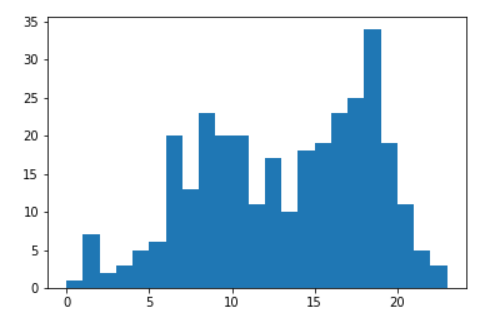在创建直方图时，有必要尝试不同的组距，看看哪个组距最能表示数据。如果组太多，可能会发现太多噪点，干扰我们发现数据里蕴含的规律。如果组太少，则根本无法看出真正的规律。
plt.figure(figsize = [10, 5]) # larger figure size for subplots

# histogram on left, example of too-large bin size
plt.subplot(1, 2, 1) # 1 row, 2 cols, subplot 1
bin_edges = np.arange(0, df['num_var'].max()+4, 4)
plt.hist(data = df, x = 'num_var', bins = bin_edges)

# histogram on right, example of too-small bin size
plt.subplot(1, 2, 2) # 1 row, 2 cols, subplot 2
bin_edges = np.arange(0, df['num_var'].max()+1/4, 1/4)
plt.hist(data = df, x = 'num_var', bins = bin_edges)

该示例通过 subplot 函数将两个图表并排地放到一起，函数的参数指定了子图的行数、列数和索引。figure() 函数在调用时传入了 “figsize” 参数，绘制尺寸更大的图纸，以便其中可以包含更多的子图。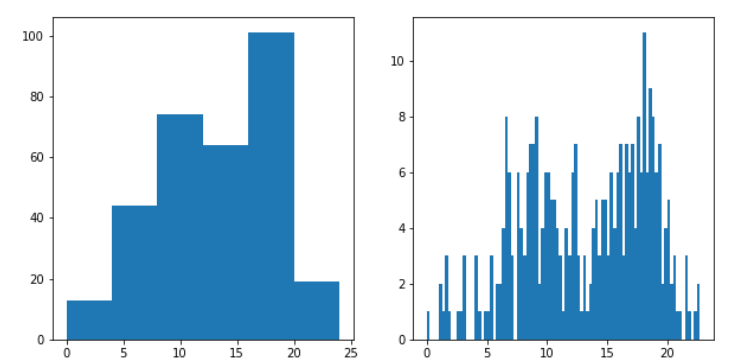其他方法
seaborn 函数 distplot 也可以用于绘制直方图，该函数集成了一些其他的单变量绘图函数
sb.distplot(df['num_var'])

注意，第一个参数_必须_是 Series 或数组，其中包含要绘制的数据点。这与我们之前看到的 countplot 和 hist 函数不同，后面两个函数需要分别指定数据源和列两个参数。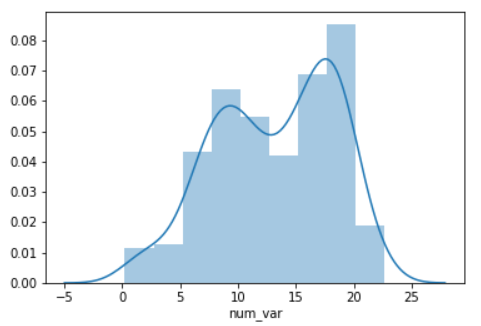distplot 函数具有绘制直方图的内置规则，默认情况下，会在数据上绘制一个 核密度估计（KDE）。纵轴基于 KDE，而不是直方图：长条的高度之和不一定等于 1，但是曲线下方的面积应该等于 1。如果你想详细了解 KDE，请参阅这节课最后 的补充内容。
虽然默认的 distplot 的分组形式比 .hist 固定的 10 个分组更好，但是你依然可能需要对它进行调整，使分组的边界值为“四舍五入”后的值。你可以使用其他参数自定义直方图，或者像之前那样指定分组：
bin_edges = np.arange(0, df['num_var'].max()+1, 1)
sb.distplot(df['num_var'], bins = bin_edges, kde = False,
hist_kws = {'alpha' : 1})

alpha（透明度）的设置必须在参数 “hist_kws” 的字典里，因为还有其他底层绘图函数（例如 KDE）具有自己的可选关键字参数。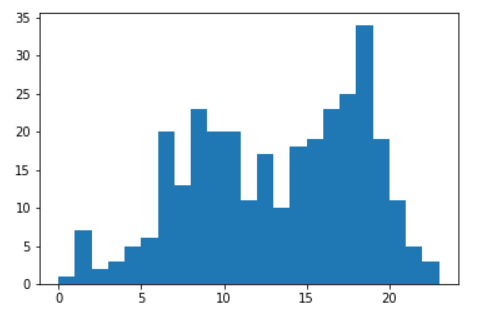总之，如果你只想了解数据的直方图分布情况，而不想要 distplot 提供的额外信息，为了简便，建议只使用 Matplotlib 的 hist 函数。另一方面，如果你想快速了解如何为直方图选择代表性的分组组距，建议在自定义之前，先快速查看下 一下distplot。
展开全文python pycharm 机器学习
• Seaborn是Python中的一个库，主要用于生成统计图形。 Volodymyr Hryshchenko在Unsplash上拍摄 ​ Seaborn是构建在matplotlib之上的数据可视化库，与Python中的pandas数据结构紧密...线性回归曲线的自动计算和绘制
Seaborn是Python中的一个库，主要用于生成统计图形。Volodymyr Hryshchenko在Unsplash上拍摄

​ Seaborn是构建在matplotlib之上的数据可视化库，与Python中的pandas数据结构紧密集成。可视化是Seaborn的核心部分，可以帮助探索和理解数据。
​ 要了解Seaborn，就必须熟悉Numpy和Matplotlib以及pandas。
​ Seaborn提供以下功能:
面向数据集的API来确定变量之间的关系。线性回归曲线的自动计算和绘制。它支持对多图像的高级抽象绘制。可视化单变量和双变量分布。
​ 这些只是Seaborn提供的功能的一部分，还有很多其他功能，我们可以在这里探索所有的功能。
​ 要引入Seaborn库，使用的命令是:
import seaborn as sns

​ 使用Seaborn，我们可以绘制各种各样的图形，如:
分布曲线饼图和柱状图散点图配对图热力图

在文章中，我们使用从Kaggle下载的谷歌Playstore数据集。

1.分布曲线
​ 我们可以将Seaborn的分布图与Matplotlib的直方图进行比较。它们都提供非常相似的功能。这里我们画的不是直方图中的频率图，而是y轴上的近似概率密度。
​ 我们将在代码中使用**sns.distplot()**来绘制分布图。
​ 在进一步之前，首先，让我们访问我们的数据集，
import pandas as pd
import numpy as np


从我们的系统访问数据集

​ 数据集是这样的，从Kaggle获得的谷歌播放商店数据集

​ 现在，让我们看看如果我们绘制来自上述数据集的“Rating”列的分布图是怎样的，
#importing all the libraries
import numpy as np
import pandas as pd
import matplotlib.pyplot as plt
import seaborn as sns

#Create a distribution plot for rating
sns.distplot(pstore.Rating)
plt.show()


Rating列分布图的代码

​ Rating列的分布图是这样的，​ 在这里，曲线(KDE)显示在分布图上的是近似的概率密度曲线。
​ 与matplotlib中的直方图类似，在分布方面，我们也可以改变类别的数量，使图更容易理解。
​ 我们只需要在代码中加上类别的数量，
#Change the number of bins
sns.distplot(inp1.Rating, bins=20, kde = False)
plt.show()

​ 图像是这样的，特定类别数的分布图

​ 在上图中，没有概率密度曲线。要移除曲线，我们只需在代码中写入**’ kde = False '**。
​ 我们还可以向分布图提供与matplotlib类似的容器的标题和颜色。让我们看看它的代码，
#importing all the libraries
import numpy as np
import pandas as pd
import matplotlib.pyplot as plt
import seaborn as sns

#Create a distribution plot for rating
sns.distplot(pstore.Rating, bins=20, color="g")
plt.title("Distribution of app ratings", fontsize=20, color = 'red')
plt.show()

​ 同一列Rating的分布图是这样的:有标题的分布图

​ 对Seaborn图形进行样式化
​ 使用Seaborn的最大优势之一是，它为图形提供了广泛的默认样式选项。
​ 这些是Seaborn提供的默认样式。
'Solarize_Light2',
'_classic_test_patch',
'bmh',
'classic',
'dark_background',
'fast',
'fivethirtyeight',
'ggplot',
'grayscale',
'seaborn',
'seaborn-bright',
'seaborn-colorblind',
'seaborn-dark',
'seaborn-dark-palette',
'seaborn-darkgrid',
'seaborn-deep',
'seaborn-muted',
'seaborn-notebook',
'seaborn-paper',
'seaborn-pastel',
'seaborn-poster',
'seaborn-talk',
'seaborn-ticks',
'seaborn-white',
'seaborn-whitegrid',
'tableau-colorblind10'

​ 我们只需要编写一行代码就可以将这些样式合并到我们的图中。
#importing all the libraries
import numpy as np
import pandas as pd
import matplotlib.pyplot as plt
import seaborn as sns

#Adding dark background to the graph
plt.style.use("dark_background")

#Create a distribution plot for rating
sns.distplot(pstore.Rating, bins=20, color="g")
plt.title("Distribution of app ratings", fontsize=20, color = 'red')
plt.show()

​ 在将深色背景应用到我们的图表后，分布图看起来是这样的，深色背景的分布图

2.饼图和柱状图
​ 饼图通常用于分析数字变量在不同类别之间如何变化。
​ 在我们使用的数据集中，我们将分析内容Rating栏中的前4个类别的执行情况。
​ 首先，我们将对内容Rating列进行一些数据清理/挖掘，并检查其中的类别。
#importing all the libraries
import numpy as np
import pandas as pd
import matplotlib.pyplot as plt
import seaborn as sns

#Analyzing the Content Rating column
pstore['Content Rating'].value_counts()

​ 类别列表是，Rating列数

​ 根据上面的输出，由于“只有18岁以上的成年人”和“未分级”的数量比其他的要少得多，我们将从内容分级中删除这些类别并更新数据集。
#importing all the libraries
import numpy as np
import pandas as pd
import matplotlib.pyplot as plt
import seaborn as sns

#Remove the rows with values which are less represented
pstore = pstore[~pstore['Content Rating'].isin(["Adults only 18+","Unrated"])]

#Resetting the index
pstore.reset_index(inplace=True, drop=True)

#Analyzing the Content Rating column again
pstore['Content Rating'].value_counts()

​ 更新后在“Rating”栏中出现的类别是:更新数据集后的Rating计数

​ 现在，让我们为Rating列中出现的类别绘制饼图。
#importing all the libraries
import numpy as np
import pandas as pd
import matplotlib.pyplot as plt
import seaborn as sns

#Plotting a pie chart
plt.figure(figsize=[9,7])
pstore['Content Rating'].value_counts().plot.pie()
plt.show()

​ 上面代码的饼状图如下所示，用于Rating的饼状图

​ 从上面的饼图中，我们不能正确的推断出“所有人10+”和“成熟17+”。当这两类人的价值观有点相似的时候，很难评估他们之间的差别。
​ 我们可以通过将上述数据绘制成柱状图来克服这种情况。
#importing all the libraries
import numpy as np
import pandas as pd
import matplotlib.pyplot as plt
import seaborn as sns

#Plotting a bar chart
plt.figure(figsize=[9,7])
pstore['Content Rating'].value_counts().plot.barh()
plt.show()

​ 柱状图如下所示，Rating栏的条形图

​ 与饼图类似，我们也可以定制柱状图，使用不同的柱状图颜色、图表标题等。
3.散点图
​ 到目前为止，我们只处理数据集中的一个数字列，比如评级、评论或大小等。但是，如果我们必须推断两个数字列之间的关系，比如“评级和大小”或“评级和评论”，会怎么样呢?
​ 当我们想要绘制数据集中任意两个数值列之间的关系时，可以使用散点图。此图是机器学习领域的最强大的可视化工具。
​ 让我们看看数据集评级和大小中的两个数字列的散点图是什么样子的。首先，我们将使用matplotlib绘制图，然后我们将看到它在seaborn中的样子。
​ 使用matplotlib的散点图
#import all the necessary libraries
#Plotting the scatter plot
plt.scatter(pstore.Size, pstore.Rating)
plt.show()

​ 图是这样的使用Matplotlib的散点图

​ 使用Seaborn的散点图
​ 在直方图和散点图的代码中，我们将使用sn .joinplot()。
​ **sns.scatterplot()**散点图的代码。
#importing all the libraries
import numpy as np
import pandas as pd
import matplotlib.pyplot as plt
import seaborn as sns

# Plotting the same thing now using a jointplot
sns.jointplot(pstore.Size, pstore.Rating)
plt.show()

​ 上面代码的散点图如下所示，使用Seaborn的散点图

​ 在seaborn中使用散点图的主要优点是，我们将同时得到散点图和直方图。
​ 如果我们想在代码中只看到散点图而不是组合图，只需将其改为**“scatterplot”**
​ 回归曲线
​ 回归图在联合图(散点图)中建立了2个数值参数之间的回归线，并有助于可视化它们的线性关系。
#importing all the libraries
import numpy as np
import pandas as pd
import matplotlib.pyplot as plt
import seaborn as sns

# Plotting the same thing now using a jointplot
sns.jointplot(pstore.Size, pstore.Rating, kind = "reg")
plt.show()

​ 图是这样的，在Seaborn中使用jointplot进行回归分析

​ 从上图中我们可以推断出，当app的价格上升时，评级会稳步上升。
4.配对图
​ 当我们想要查看超过3个不同数值变量之间的关系模式时，可以使用配对图。例如，假设我们想要了解一个公司的销售如何受到三个不同因素的影响，在这种情况下，配对图将非常有用。
​ 让我们为数据集的评论、大小、价格和评级列创建一对图。
​ 我们将在代码中使用**sns.pairplot()**一次绘制多个散点图。
#importing all the libraries
import numpy as np
import pandas as pd
import matplotlib.pyplot as plt
import seaborn as sns

# Plotting the same thing now using a jointplot
sns.pairplot(pstore[['Reviews', 'Size', 'Price','Rating']])
plt.show()

​ 上面图形的输出图形是这样的，使用Seaborn的配对图

对于非对角视图，图像是两个数值变量之间的散点图  对于对角线视图，它绘制一个柱状图，因为两个轴(x,y)是相同的。
5.热力图
​ 热图以二维形式表示数据。热图的最终目的是用彩色图表显示信息的概要。它利用了颜色强度的概念来可视化一系列的值。
​ 我们在足球比赛中经常看到以下类型的图形，足球运动员的热图

​ 在Seaborn中创建这个类型的图。
​ 我们将使用**sn .heatmap()**绘制可视化图。
​ 当你有以下数据时，我们可以创建一个热图。上面的表是使用来自Pandas的透视表创建的。

​ 现在，让我们看看如何为上表创建一个热图。
#importing all the libraries
import numpy as np
import pandas as pd
import matplotlib.pyplot as plt
import seaborn as sns

##Plot a heat map
sns.heatmap(heat)
plt.show()

​ 在上面的代码中，我们已经将数据保存在新的变量“heat”中。
​ 热图如下所示，使用Seaborn创建默认热图

​ 我们可以对上面的图进行一些自定义，也可以改变颜色梯度，使最大值的颜色变深，最小值的颜色变浅。
​ 更新后的代码是这样的，
#importing all the libraries
import numpy as np
import pandas as pd
import matplotlib.pyplot as plt
import seaborn as sns

#Applying some customization to the heat map
sns.heatmap(heat, cmap = "Greens", annot=True)
plt.show()

​ 上面代码的热图是这样的，带有一些自定义的热图代码

​ 在我们给出**“annot = True”的代码中，当annot为真时，图中的每个单元格都会显示它的值。如果我们在代码中没有提到annot**，那么它的默认值为False。
​ Seaborn还支持其他类型的图形，如折线图、柱状图、堆叠柱状图等。但是，它们提供的内容与通过matplotlib创建的内容没有任何不同。
结论
​ 这就是Seaborn在Python中的工作方式以及我们可以用Seaborn创建的不同类型的图形。正如我已经提到的，Seaborn构建在matplotlib库之上。因此，如果我们已经熟悉Matplotlib及其函数，我们就可以轻松地构建Seaborn图并探索更深入的概念。
​ 感谢您的阅读!!
作者：Kaushik Katari
deephub翻译组：孟翔杰
展开全文可视化 python 数据分析
• # 饼图 plt . pie ( x , label , explode , shadow = True , pctdistance = 0.6 , labeldistance = 1.1 ，startangle = 90 ) # explode表示部分扇形突出，x,label,explode均为数组形式数据 # 极坐标图 ax =...
基础图形
针对各种基本图形，seaborn中提供了与matplotlib类似的接口，其他高级作图函数都以这些底层作图函数为基础，进行封装，通常作为kind参数。各种基本图形既可以直接传入数组形式的变量数据，也可以传入DataFrame列名并传入data参数。 列举如下：
import seaborn as sns
# 折线图
sns.lineplot()
# 条形图
sns.barplot()
# 计数条形图
sns.countplot()
# 散点图
sns.scatterplot()
# 分类散点图
sns.stripplot()
# 分簇散点图
sns.swarmplot() # 与stipplot()的区别就是点不重叠
# 箱型图
sns.boxplot()
# 增强箱型图
sns.boxenplot() # 适合大数据集，显示更多分位数
# 小提琴图
sns.violinplot()
# 点图
sns.pointplot() # 纵轴是均值，置信区间用标准差表示
# 核密度估计图
# 地毯图
sns.rugplot(x) # 直接将数据标记在坐标轴上
# 回归线图
sns.regplot() # 散点图附加回归线
# 热图
sns.heatmap(annot=True) # annot表示显示数值
# 另外补充几种常用图
# 饼图
# 极坐标图
ax = plt.subplot(111,projection='polar') # projection指投影到极坐标
ax.plot(x,y) # x为角度(弧度制),y为径长
# 平行坐标图
from pandas.plotting import parallel_coordinates
parallel_coordinates(data, 'key')
高级函数
针对数据可视化的不同目的，seaborn提供了relplot()，catplot()，displot()，lmplot()四大主要高级函数。 seaborn与pandas的DataFrame的结合非常好，因此传参是可以直接传入列名。 返回的是FacetGrid(平面网格图)对象。 这些高级函数的主要参数如下： x，y：输入变量 data：输入数据df hue：分组变量 style：如传入，则hue分组后每组数据作图风格可不一致 col，row：决定平面网格图布局的变量 kind：底层作图类型名称，如"line",“bar”,"box"等 注：由于返回的平面网格图子图间距可能不合理，可调用plt.tight_layout()自动调整。
可视化变量关系(relationship)
relplot()方便观察变量间的关系。
sns.relplot() # 默认是散点图
分类数据的分布图(categorical)
catplot()可以很好观察分类数据的分布情况。
sns.catplot()
数据集分布图(distribution)
特征工程前，需要对数据集的有一个整体的了解，seabon提供的高级函数displot()可以作各种分布图。
单变量分布
displot是hist(直方图)、rugplot(地毯图)、kdeplot(核密度估计图)的高级封装。
from scipy import stats
sns.displot(x, kde=False,rug=False, fit=stats.gamma) # 直方图,若传入fit参数，则表示拟合相应的分布
双变量分布
sns.jointplot(kind='scatter')
# scatter表示散点图，hex表示HexBin图，kde表示核密度估计图，reg表示添加回归线的散点图，resid表示残差图
# 定制图
g = sns.JointGrid()
g.plot_joint(plt.scatter)
g.plot_marginal(sns.displot)
g.annotate(stats.pearsonr)
成对变量分布
sns.pairplot(data)
# 定制图
g = sns.PairGrid(data)
g.map_diag(sns.kdeplot)
g.map_offdiag(sns.kdeplot)
回归线图(linear model)
regplot()以及lmplot()都可以作回归线图，二者的区别是lmplot的参数data是必传的，而regplot不是。因此可以将regplot()视为底层函数。
sns.lmplot('total_bill','tip',data=tips)
sns.residplot() # 残差图
"""
lmplot重要参数解释：
ci:置信度
order: 拟合阶数
x_jitter: 施加噪声
robust: 鲁棒性
logistic: 逻辑斯谛回归
hue：分组回归
scatter_kws={"s":80}: 散点图参数(例如点的大小)
"""
展开全文• ## seaborn画饼图

万次阅读 2017-12-10 16:29:50
(一)饼图：1）用到的方法：matplotlib.pyplot.pie() 2）参数解析：pie(x, explode=None, labels=None, colors=('b', 'g', 'r', 'c', 'm', 'y', 'k', 'w'), autopct=None, pctdistance=0.6, shadow=False, ...
(一)饼图：
1）用到的方法：matplotlib.pyplot.pie()  2）参数解析：
pie(x, explode=None, labels=None,
colors=('b', 'g', 'r', 'c', 'm', 'y', 'k', 'w'),
counterclock=True, wedgeprops=None, textprops=None,
center = (0, 0), frame = False )

3）参数说明：

x (每一块)的比例，如果sum(x) > 1会使用sum(x)归一化 labels (每一块)饼图外侧显示的说明文字  explode (每一块)离开中心距离 startangle 起始绘制角度,默认图是从x轴正方向逆时针画起,如设定=90则从y轴正方向画起  shadow 是否阴影 labeldistance label绘制位置,相对于半径的比例, 如<1则绘制在饼图内侧 autopct  控制饼图内百分比设置,可以使用format字符串或者format function  ‘%1.1f’指小数点前后位数(没有用空格补齐) pctdistance 类似于labeldistance,指定autopct的位置刻度 radius 控制饼图半径
返回值: 如果没有设置autopct,返回(patches, texts) 如果设置autopct,返回(patches, texts,  autotexts) patches – list –matplotlib.patches.Wedge对象 texts  autotexts – matplotlib.text.Text对象

（二）distplot图：
1）解析：  seaborn的displot()集合了matplotlib的hist()与核函数估计kdeplot的功能，增加了rugplot分布观测条显示与利用scipy库fit拟合参数分布的新颖用途  2）用法：
seaborn.distplot(a, bins=None, hist=True, kde=True, rug=False, fit=None, hist_kws=None, kde_kws=None, rug_kws=None, fit_kws=None, color=None, vertical=False, norm_hist=False, axlabel=None, label=None, ax=None)
3)参数说明：
a:观察数据，一维的或者是数组。
如果是Series对象并且带有name属性，name就会作为标记一个数据轴。
bins:设置矩形数量。
hist:bool,可选。控制是否显示条形图。
kde:bool,optional.控制是否显示核密度图。
rug:随机变量，可选。控制拟合的参数分布图。
vertical:bool,可选。显示正交控制。
展开全文• nums = [20, 36, 40, 37, 6] labels = ['High-school','Bachelor','Master','Ph.d', 'Others'] # 用 Matplotlib 绘制饼图 plt.pie(x = nums, labels=labels) plt.show() 图形如下 热力图， 热力图英文名叫heat map，...
• 在 Matplotlib 中，我们使用 plt.pie(x, labels=None) 函数，其中参数 x 代表要绘制饼图的数据，labels 是缺省值，可以为饼图添加标签。 # data 7.热力图 热力图，英文叫 heat map，是一种矩阵表示方法，其中矩阵中...
• 在使用Matplotlib 绘制饼图的时候有些时候一些数据的比列太小在饼图呈现的效果不明显 很容易被覆盖，为了解决这个问题以下就是我个人的心得。 【未解决之前呈现的效果】 可以看到这个饼状图其他和硕士这2个部分...
• 能来到这篇文章的对 matplotlib 或者 seaborn 应该都挺熟的???? 所以直接上代码和效果图 import matplotlib.pyplot as plt # make the pie circular by setting the aspect ratio to 1 # plt.figure(figsize=plt....python 数据分析 可视化 数据可视化
• Seaborn是一个基于matplotlib的Python可视化库。 它提供了一个高级界面，用于绘制有吸引力的统计图形
• 点击上方“Python爬虫与数据挖掘”，进行关注回复“书籍”即可获赠Python从入门到进阶共10本电子书今日鸡汤风朝露夜阴晴里，万户千门开闭时。Seaborn简介定义Seaborn是一...可视化 数据可视化 less python ebook
• 由于matplotlib比较底层，想要绘制漂亮的图非常麻烦，需要写大量的代码。 Seaborn是在matplotlib基础上进行了高级API封装，图表装饰更加容易，你可以用更少的代码做出更美观的图。同时，Seaborn高度兼容了numy、...
• 使用python的seaborn绘制折线图与柱状图的组合图前言代码结果 前言 今天入职，小组长给我们布置了数据可视化的作业，让大家浏览一个可视化系统，然后找到三个结论，其实很简单，但是自己又拓展一点。然后需要画一个...python可视化 matplotlib
• 但在地图上绘制饼图时，它也有自己的缺点。首先，当我们绘制大量的饼图时，它以光栅图像的形式渲染，使得它的渲染速度很慢。 本文创建了一个封装函数，使其更容易绘制一组饼图。 例如，假设我们有以下数据。 set....R语言 地图 可视化
• ## Python实现饼形图的绘制

万次阅读 多人点赞 2018-11-08 10:58:58
说明：代码运行环境为 Win10+Python3+jupyter notebook ...绘制饼形图的主要方法： 方法1：利用pandas中的Series.plot()或DataFrame.plot.pie()方法 方法2：利用matplotlib包中的调用axes.pie()方法 首先导出需要...Python可视化 数据分析
• Top 50 matplotlib Visualizations – The Master Plots (with full python code) ...import seaborn as sns import pandas as pd import numpy as np import matplotlib.pyplot as plt import warn...python matplotlib
• Seaborn 散点 pyplot.scatter jointplot(x=’’,y=’’,data=,kind=‘scatter’) 折线 pyplot.plot(x,y) lineplot(x=’’,y=’’,data=) 直方图 pyplot.hist(x,bin=) distplot(x,kde=True) 条形图 pyplot....
• http://seaborn.pydata.org/tutorial/color_palettes.html import numpy as np import pandas as pd import matplotlib.pyplot as plt %matplotlib inline # 绘图，figsize设置大小 def sinplot(): x = ...python
• 绘制各班级人数饼图 explode=[0,0.1,0,0.2] # 设置每个扇形弹出的距离 colors = ['red','yellow','blue','green'] # 设置每个扇形颜色 # 绘制饼图，labels是班级名称，atuopct是百分比显示格式，pctdistance显示距离...python
• 1 饼图知识 饼图：用于表示不同分类的占比情况，通过弧度大小来对比各种分类。 特点：分类数据的占比情况(占比) 饼图广泛得应用在各个领域，用于表示不同分类的占比情况，通过弧度大小来对比各种分类。饼图通过将一...python matplotlib 分类数据
• 利用Pandas及Seaborn库，并使用Pandas及Seaborn结合Numpy 及Matplotlib绘制以下可视化图 折线图 饼图 横向柱状图 纵向柱状图 散点图 气泡图 直方图python
• df/s.plot() data = pd.read_csv() Series.boxplot() data.plot( kind='', 无kind则默认折线图 logy, y轴上是否转化为log ... ax, 绘制的图的子图位置 color, style, 颜色、标记、线形 alpha, ...
• 本文的文字及图片来源于网络,仅供学习、交流使用,不具有任何商业用途,版权归原作者所有,如有问题请及时联系我们以作处理 ...今天我们就用五种方法来绘制柱状图，分别是matplotlib，seaborn，plotnine，pye可视化 python 数据可视化 数据分析
• 优势在哪里？使用Matplotlib通过iris.Name.unique()可以看出有三种花代码结果通过Seaborn去画只需要一行代码 直方图和密度图（matplotlib）直方图密度图Seaborn中各种图：kde表示密度图 ...
• 完整的代码以及Python可视化指南，介绍了Pandas，Seaborn和Plotly的绘图。 2018: Regplot showing how Life Ladder (Happiness) is positively correlated with Log GDP per capita ( 在今天的文章中，我们将研究...python 数据可视化
• seaborn 上面有很多炫酷的案例可供参考使用 其实很多属性与绘制折线图有很多重复的地方,参考上篇折线图. 散点图 """散点图""" from matplotlib import pyplot as plt from matplotlib import font_manager my_font...数据可视化
• 概况： 1、pymysql连接数据库 ...5、获取饼图，先pd.value_counts对关键列表计数去重，得到新的表格后， 获取index作为标签 获取列data作为数据index=result.index.tolist() data=result.data.tolist() matplotli..python mysql matplotlib
• using the squarify package (inspired by a post on how to do treemaps): import numpy as np import pandas as pd import matplotlib as mpl import matplotlib.pyplot as plt import seaborn as sns # ...
• 这是一份关于seaborn 和matplotlib 的进阶笔记，我个人觉得画图还是用seaborn，相对更简单上手一点。这里面的函数我还有很多没找到，有些我也不是很知道里面参数具体有什么，能干什么，不过这些基本操作也能对你我...机器学习 可视化 python matplotlib
• 1. 写在前面 今天的这篇文章是继快速入门数据分析系列的第四篇...Python进行可视化分析的库有matplotlib和SeabornSeaborn相当于matplotlib更加高级的一个库，两者的关系类似于numpy与pandas的关系（后者比前者...数据分析基础...